# Affine Cipher

In the section on Caesar ciphers, we saw that there are only 26 possible ways of encrypting a message in English when using a Caesar cipher. The Affine cipher is an attempt to improve on this. The end result, though, is a cipher that a computer can still crack without even really trying. The 26 possible Caesar shifts are replaced with 676 (i.e. 26 x 26) Affine shifts, and 676 is still a trivially small number of possibilities for a computer to work through. Note: the number of possibilities is actually fewer than 676, as we shall see ...

#### How the Affine cipher works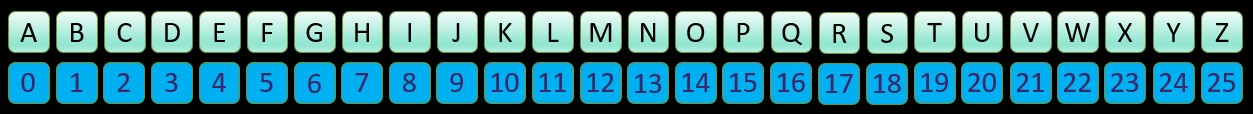As with a Caesar cipher, it is helpful to think of each letter having a number in the range 0-25, as shown in the image above.

The Caesar cipher applied a simple shift (e.g. +4) to the number of each plaintext letter to give the number of the ciphertext letter. If the new number was greater than 25, the cipher then required you to wrap around back to the start of the alphabet. So, for example, a Caesar shift of +4 on 'Y' (i.e. 24) would give a number of 28, which became the number 2 when wrapping back around to the start of the alphabet.

The Affine cipher is only a little more complicated:

• The Caesar cipher has 1 number in its key: the shift size.
• The Affine has two numbers in its key: a multiplier + a shift size.

Here is an example to see how the two parts in an Affine cipher key are used. We will encrypt 'affine cipher' using a multiplier of 3 followed by a shift of 11: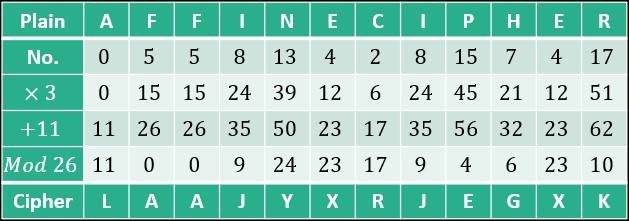Most of the table is self-explanatory, except the penultimate 'Mod 26' row. The Affine cipher takes the number of a plain text letter, multiplies it by a certain number, and then adds on the shift size. This can generate some quite large numbers. If, for example, the multiplier was 25, the shift size was 9 and the plain text letter was 'Y' (i.e. 24), then the final number is (25 x 24) + 9, i.e. 609. As with the Caesar cipher, we need to wrap around to the start of the alphabet, but, because 609 is so large, we will need to do this multiple times. The quick way to do this is find the remainder when 609 is divided by 26. This is known as modular division and more information about it can be found here.

#### A catch ...

One problm with the Affine cipher can be seen in the following table. The phrase 'affine cipher' has been encoded using a multiplier of 2 and a shift size of 11.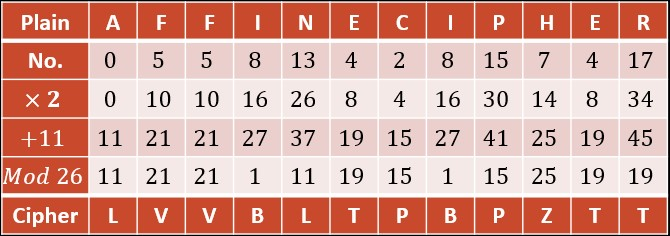The problem with this key is that both the 'A' and 'N' in 'affine' have been turned into 'L', and both the 'E' and 'R' in 'cipher' have been turned into 'T'. This creates a problem for the intended recipient of the encrypted message: if they see a 'T' in the ciphertext, they will not know whether it is meant to be an 'E' or an 'R', and they will have similar problems if they see the letter 'L'. If the word 'rear' had occurred in the plaintext, then this would have been turned into 'TTLT'! It's all very well enciphering a message into something confusing, but not if this means that even the intended recipient cannot make any sense of it.

#### Working out what has gone wrong

To work out what has gone wrong, we will use an even simpler key: multiplier = 2, shift = 0.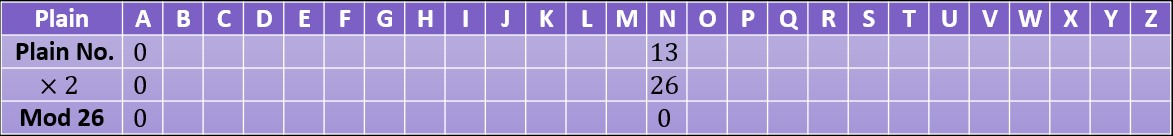Because 'a' is the number 0, then, when we multiply it by the multiplier (i.e. 2), the outcome will stay as 0.

The multiplier, 2, is a factor of 26. This means that there is another number between 0 and 25, which, when multiplied by 2, equals 26. In this case, that number is obviously 13.

When we then turn to the letter that has that second number of 13, i.e. the letter 'n', and then multiply it by our multiplier of 2, then we will get 26. When we apply modular division by 26 to 26, we end up with 0. The letter 'n', therefore, will end up the same as the letter 'a' because, as we just noted, 'a' also ends up as 0.

But it gets worse ...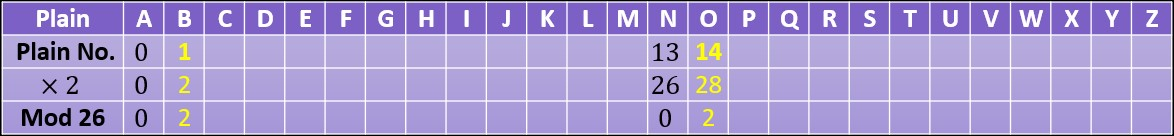The gap between 'a' and 'n', after being multiplied by 2, is a multiple of 26. In this case, the gap is exactly 1 x 26, or 26. Only if the gap is exactly a multiple of 26 could they end up with the same value when modular division by 26 is applied. With this in mind, now think about the gap between the next two letters, 'b' and 'o'. The move from 'a' to 'b' is the move from (0 x 2) to (1 x 2), whereas the move from 'n' to 'o' is the move from (13 x 2) to (14 x 2). In both cases, the new output is 2 more than the previous letter. But, because the previous two letters of 'a' and 'n' shared the same outcome when modular division by 26 was applied (i.e. 0), then these next two numbers, being both 2 more, are going to give a remainder of 2 when divided by 26. Hence they will end up becoming the same ciphertext letter ('c').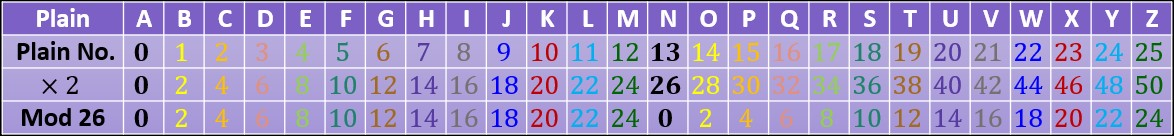This problem repeats as we move to the next two letters, 'c' and 'p', then 'd' and 'q', and so on.

To avoid this problem, we must pick a multiplier such that when we look at the penultimate row in these tables (i.e. the multiplier rows), then no two numbers in that row should ever be separated by an exact multiple of 26. A separation of an exact multiple of 26 will happen if the multiplier shares a prime factor with 26. We can never, therefore, pick an even number as the multiplier, because they share the prime factor of 2 with 26, and we can also not pick 13, because it shares the prime factor of 13 with 26. The only legitimate multipliers, therefore, are as follows: 1, 3, 5, 7, 9, 11, 15, 17, 19, 21, 23, 25.

But what about multipliers higher than 25, such as 27? 27 shares no prime factors with 26, so can we use it? Yes, we can, but it ends up being the same as a multiplier of 1.

If you multiply a number n by 27, then it is the same as this: (26 x n) + (1 x n).

The total, therefore is greater than (1 x n) by the amount of (26 x n). But this difference is a multiple of 26 which means, that when we add on this total and wrap around the alphabet, we will end up exactly where we started, i.e. at the position of (1 x n). Adding on a multiple of 26 makes no difference, therefore, when you apply modular division by 26. Hence a multiplier of 27 is the same as a multiplier of 1.

By the same logic, a multiplier of 28 is the same as a multiplier of 2. A multiplier of 29 is the same as a multiplier of 3, and so on.

The problem that I have been describing here is all caused by the multiplier sharing a factor with 26. The size of the shift, the second part of the Affine cipher, makes no difference. The problems come when, after applying the multiplier, the gap between any two letters in the table is a multiple of 26. Applying a shift size after applying the multiplier makes no difference to the size of these gaps between letters - the shift preserves the gap sizes that are created after the multiplier has been applied.

All the shift sizes between 0 and 25, therefore, are permissible. Going higher than 25 is pointless because the shift will just wrap back around to the start, i.e. a shift size of 26 is the same as a shift size of, a shift of 27 is the same as a shift of 1 etc.

So, for a 26 letter alphabet, we have the 12 possible multipliers listed above, followed by 26 possible shift sizes. Total number of possible keys: 12 x 26 = 312.

Cipher Challenge competition    Leave feedback